# WO2011102472A1 - Battery state estimation device and method of estimating battery state - Google Patents

Battery state estimation device and method of estimating battery state Download PDF

## Info

Publication number
WO2011102472A1
WO2011102472A1 PCT/JP2011/053534 JP2011053534W WO2011102472A1 WO 2011102472 A1 WO2011102472 A1 WO 2011102472A1 JP 2011053534 W JP2011053534 W JP 2011053534W WO 2011102472 A1 WO2011102472 A1 WO 2011102472A1
Authority
WO
WIPO (PCT)
Prior art keywords
voltage
battery
measurement value
value
secondary battery
Prior art date
Application number
PCT/JP2011/053534
Other languages
French (fr)
Japanese (ja)
Inventor

Original Assignee

Priority date (The priority date is an assumption and is not a legal conclusion. Google has not performed a legal analysis and makes no representation as to the accuracy of the date listed.)
Filing date
Publication date
Priority to JP2010033903 priority Critical
Priority to JP2010-033903 priority
Priority to JP2011-026032 priority
Priority to JP2011026032A priority patent/JP5691592B2/en
Application filed by 日産自動車株式会社 filed Critical 日産自動車株式会社
Priority claimed from RU2012108577/28A external-priority patent/RU2491566C1/en
Publication of WO2011102472A1 publication Critical patent/WO2011102472A1/en

• 238000001914 filtration Methods 0.000 claims abstract description 18
• 238000004364 calculation methods Methods 0.000 claims description 43
• 230000003044 adaptive Effects 0.000 claims description 28
• 238000006243 chemical reaction Methods 0.000 claims description 25
• 238000000034 methods Methods 0.000 claims description 17
• 230000000694 effects Effects 0.000 description 9
• 238000004088 simulation Methods 0.000 description 5

## Images

•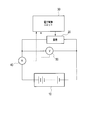•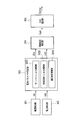•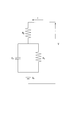•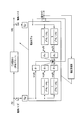•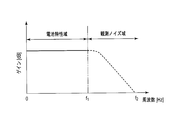•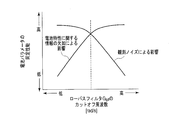•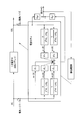•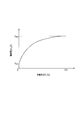•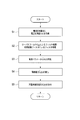•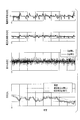•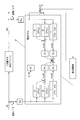•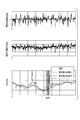•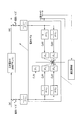•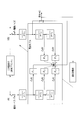## Classifications

• HELECTRICITY
• H01BASIC ELECTRIC ELEMENTS
• H01MPROCESSES OR MEANS, e.g. BATTERIES, FOR THE DIRECT CONVERSION OF CHEMICAL ENERGY INTO ELECTRICAL ENERGY
• H01M10/00Secondary cells; Manufacture thereof
• H01M10/42Methods or arrangements for servicing or maintenance of secondary cells or secondary half-cells
• H01M10/48Accumulators combined with arrangements for measuring, testing or indicating condition, e.g. level or density of the electrolyte
• H01M10/482Accumulators combined with arrangements for measuring, testing or indicating condition, e.g. level or density of the electrolyte for several batteries or cells simultaneously or sequentially
• GPHYSICS
• G01MEASURING; TESTING
• G01RMEASURING ELECTRIC VARIABLES; MEASURING MAGNETIC VARIABLES
• G01R31/00Arrangements for testing electric properties; Arrangements for locating electric faults; Arrangements for electrical testing characterised by what is being tested not provided for elsewhere
• G01R31/36Arrangements for testing, measuring or monitoring the electrical condition of accumulators or electric batteries, e.g. capacity or state of charge [SoC]
• G01R31/382Arrangements for monitoring battery or accumulator variables, e.g. SoC
• G01R31/3842Arrangements for monitoring battery or accumulator variables, e.g. SoC combining voltage and current measurements
• GPHYSICS
• G01MEASURING; TESTING
• G01RMEASURING ELECTRIC VARIABLES; MEASURING MAGNETIC VARIABLES
• G01R31/00Arrangements for testing electric properties; Arrangements for locating electric faults; Arrangements for electrical testing characterised by what is being tested not provided for elsewhere
• G01R31/36Arrangements for testing, measuring or monitoring the electrical condition of accumulators or electric batteries, e.g. capacity or state of charge [SoC]
• G01R31/367Software therefor, e.g. for battery testing using modelling or look-up tables
• HELECTRICITY
• H01BASIC ELECTRIC ELEMENTS
• H01MPROCESSES OR MEANS, e.g. BATTERIES, FOR THE DIRECT CONVERSION OF CHEMICAL ENERGY INTO ELECTRICAL ENERGY
• H01M10/00Secondary cells; Manufacture thereof
• H01M10/05Accumulators with non-aqueous electrolyte
• H01M10/052Li-accumulators

## Abstract

Provided are a battery state estimation device and a method of estimating a battery state wherein a parameter of a rechargeable battery is identified with high accuracy. The current and the terminal voltage of the rechargeable battery are detected, the terminal voltage of a rechargeable battery is estimated based on a given battery model using the detected current and the measured value of the terminal voltage, and the parameter of the rechargeable battery is identified such that the difference between the measured value of the terminal voltage and the estimated value of the terminal voltage converges to zero. When identifying the parameter of the rechargeable battery, filtering of the measured value of the terminal voltage and the estimated value of the terminal voltage is applied respectively thereon using a low pass filter having the common high frequency breaking characteristics, and the measured value of the terminal voltage and the estimated value of the terminal voltage to which the filtering was applied are used.

## Description

Battery state estimation device and battery state estimation method

The present invention relates to a battery state estimation device and a battery state estimation method for estimating a state inside a secondary battery.

Japanese Patent Laid-Open No. 2003-185719 discloses a control device for a secondary battery as follows. That is, the secondary battery control device defines a predetermined battery model, and converts the measured values of the current and terminal voltage of the secondary battery into state quantities using a state variable filter based on the battery model. The secondary battery control device uses this to estimate the terminal voltage of the secondary battery based on the battery model. Then, the secondary battery control device identifies the parameters of the secondary battery so that the difference between the measured voltage value and the terminal voltage estimated based on the battery model converges to zero.

However, in Japanese Patent Laid-Open No. 2003-185719, a part of the measured value of the secondary battery current and the measured value of the terminal voltage is used for the terminal voltage estimation calculation without being filtered by the state variable filter. It is done. Here, since the measured value of the current of the secondary battery and the measured value of the terminal voltage are measured by an ammeter or a voltmeter, they usually include observation noise. For this reason, Japanese Patent Laid-Open No. 2003-185719 has a problem that the identification accuracy of the parameters of the secondary battery becomes insufficient due to the influence of the observation noise.

The problem to be solved by the present invention is to provide a battery state estimation device and a battery state estimation method capable of identifying a parameter of a secondary battery with high accuracy.

In order to solve the above-described problem, an aspect of the present invention detects a current and a terminal voltage of a secondary battery, and uses a measured value of the detected current and a terminal voltage to make a terminal of the secondary battery based on a predetermined battery model. A battery state estimation device and a battery state estimation method for estimating a voltage and identifying a parameter of a secondary battery so that a difference between a terminal voltage measurement value and a terminal voltage estimation value converges to zero. When identifying the parameters of the secondary battery, the measured terminal voltage and the estimated terminal voltage are each filtered using a low-pass filter having the same high-frequency cutoff characteristics, and the filtered terminal voltage The measured value and the estimated terminal voltage are used.

According to the aspect of the present invention, when identifying the parameters of the secondary battery, the measured value of the terminal voltage and the estimated value of the terminal voltage are each subjected to the filtering process by the low-pass filter having the same high-frequency cutoff characteristic, The measured terminal voltage and the estimated terminal voltage are used. Thereby, the influence of the observation noise contained in the measured values of current and terminal voltage can be effectively removed, and as a result, the parameters of the secondary battery can be identified with high accuracy.

FIG. 1 is a diagram illustrating a configuration of a control system for a secondary battery according to the present embodiment. FIG. 2 is a functional block diagram of the electronic control unit 30 according to the present embodiment. FIG. 3 is a diagram showing an equivalent circuit model showing a battery model of the secondary battery. FIG. 4 is a configuration diagram of the adaptive identification system according to the first embodiment. FIG. 5 is a diagram illustrating frequency band characteristics of the current measurement value I (k), the voltage measurement value V (k), and the voltage estimation value V ^ (k). FIG. 6 is a correlation diagram showing the relationship between the cut-off frequency of the low-pass filter G lpf , the lack of information necessary for obtaining battery characteristics, and the effect of observation noise on battery parameter identification performance. FIG. 7 is a configuration diagram of the adaptive identification system corresponding to the equivalent conversion of FIG. FIG. 8 is a diagram illustrating an example of an open circuit voltage-charge rate characteristic of the secondary battery. FIG. 9 is a flowchart showing the estimation process of the battery parameters and the charging rate in the present embodiment. FIG. 10 is a diagram illustrating a simulation result of the charging rate estimation process according to the first embodiment. FIG. 11 is a configuration diagram of an adaptive identification system according to the second embodiment. FIG. 12 is a diagram illustrating a simulation result of the charging rate estimation process in the second embodiment. FIG. 13 is an example of a configuration diagram of an adaptive identification system according to the third embodiment. FIG. 14 is another example of a configuration diagram of the adaptive identification system according to the third embodiment.

Hereinafter, embodiments of the present invention will be described with reference to the drawings.

<< First Embodiment >>
The control system shown in FIG. 1 is applied to the present invention in a system that drives a load such as a motor with a secondary battery or charges a secondary battery with electric power generated by motor regeneration or power generated by an alternator using an engine as a power source. This is an example in which such a secondary battery control device is applied.

The secondary battery 10 is formed by connecting a plurality of unit batteries in series. Examples of the unit battery constituting the secondary battery 10 include a lithium secondary battery such as a lithium ion secondary battery. An example of the load 20 is a motor.

The current sensor 40 is a sensor that detects a charge / discharge current flowing through the secondary battery 10. A signal detected by the current sensor 40 is sent to the electronic control unit 30. The voltage sensor 50 is a sensor that detects the terminal voltage of the secondary battery 10. A signal detected by the voltage sensor 50 is sent to the electronic control unit 30.

The electronic control unit 30 is a control unit for controlling the secondary battery 10 and includes a CPU that calculates a program, a microcomputer that includes a ROM and RAM that stores programs and calculation results, an electronic circuit, and the like. .

2, the electronic control unit 30 includes a current detection unit 301, a voltage detection unit 302, a battery parameter estimation unit 303, an open circuit voltage estimation unit 304, and an SOC estimation unit 305. The battery parameter estimation unit 303 includes a low-pass filter calculation unit 3031, a state variable filter calculation unit 3032, and an adaptive identification calculation unit 3033.

The current detection unit 301 acquires a signal from the ammeter 40 at a predetermined cycle, and detects a charge / discharge current flowing through the secondary battery 10 based on the signal from the ammeter 40, thereby measuring a current measurement value I (k). To get. The current detection unit 301 sends the acquired current measurement value I (k) to the battery parameter estimation unit 303.

The voltage detection unit 302 acquires a signal from the voltmeter 50 at a predetermined cycle, and acquires a voltage measurement value V (k) by detecting a terminal voltage of the secondary battery 10 based on the signal from the voltmeter 50. To do. The voltage detection unit 302 sends the acquired current measurement value V (k) to the battery parameter estimation unit 303.

The battery parameter estimation unit 303 defines a battery model of the secondary battery 10, and uses the current measurement value I (k) detected by the current detection unit 301 and the voltage measurement value V (k) detected by the voltage detection unit 302. The battery parameters φ ^ (k) of the battery model of the secondary battery 10 are collectively estimated by adaptive digital filter calculation.

Here, “^” attached to the right shoulder in φ ^ (k) indicates that the value is an estimated value. In FIG. 2, “^”, which is an estimated value, is directly above “φ” of φ (k), directly above “V” of V0 (k), and “S” of SOC (k). As shown in the following formula (1), this is synonymous with φ ^ (k), V 0 ^ (k), and SOC ^ (k). The same applies to V ^ (k) below.

Hereinafter, a method for estimating the battery parameter φ ^ (k) of the secondary battery 10 by the battery parameter estimation unit 303 will be described.

First, the “battery model” used in the present embodiment will be described. FIG. 3 is an equivalent circuit model showing a battery model of the secondary battery 10, and the equivalent circuit model shown in FIG. 3 is expressed by the following equation (2).

Here, the model input is current I [A] (positive value is charging, negative value is discharging), model output is terminal voltage V [V], R 1 [Ω] is charge transfer resistance, R 2 [Ω] Is a pure resistance, C 1 [F] is an electric double layer capacitance, and V 0 [V] is an open circuit voltage. In the above formula (2), s is a differential operator. Note that the battery model according to the present embodiment is a reduction model (primary) in which the positive electrode and the negative electrode are not particularly separated, but it is possible to show the actual charge / discharge characteristics of the battery relatively accurately. As described above, in the present embodiment, a configuration in which the order of the battery model is first will be described as an example.

When R 1 , R 2 , and C 1 are expressed as in the following formula (3), the above formula (2) is expressed by the following formula (4).

And in this embodiment, the battery parameter estimation part 303 estimates the battery parameter (phi) ^ (k) of the battery model shown in FIG. 3 by an adaptive digital filter from the battery model shown by said Formula (4). Hereinafter, a method for estimating the battery parameter φ ^ (k) by the battery parameter estimation unit 303 will be described.

First, it is considered that the open circuit voltage V 0 (t) is obtained by integrating a current I (t) multiplied by a variable parameter h from an initial state. In this case, the open circuit voltage V 0 (t) can be expressed by the following formula (5).

When the above formula (5) is substituted into the above formula (4), the following formula (6) is obtained, and when this is arranged, the following formula (7) is obtained.

The above formula (2) and the above formula (7) correspond to the following formula (8) and the following formula (9), respectively. In the following formula (8) and the following formula (9), A (s), This corresponds to the order of B (s) being the primary.

Here, A (s) and B (s) are polynomial functions of s, and A (s) and B (s) have the same order.

Then, by introducing known constants k i (i = 1, 2,..., N) into the above formula (7), the following formulas (10) and (11) can be obtained.

In the above equation (11), I i and b 0i are parameters including unknown parameters (T 1 , T 2 , K, h). f Vi and f Ii are converted state quantities obtained by filtering I (k) and V (k), which are values measurable by the ammeter 40 and the voltmeter 50, with a state variable filter. And since said Formula (11) is these product sum formulas, it corresponds with following formula (12) which is a standard form of an adaptive digital filter.

However, in the above formula (12), φ T = [I i , b 0i ] and ω = [f Vi , f Ii ].

Then, from the converted state quantity ω (k), a voltage estimated value V ^ (k) that is an estimated value of the terminal voltage of the secondary battery 10 estimated from the battery model described above, and a voltage detected by the voltmeter 50 Based on the algorithm shown in the following formula (13) by the adaptive adjustment rule so that the difference between the actual measured value acquired by the detecting unit 302 and the voltage measured value V (k) converges to zero. The battery parameter φ ^ (k) of the model is identified. In this case, in the present embodiment, it is possible to use a “both-end trace gain method” in which a logical defect of a simple “adaptive digital filter based on the least square method” is improved. Note that the above-mentioned logical drawback is that once the estimated value converges, accurate estimation cannot be performed again even if the parameter changes thereafter.

The above equation (13) is a sequential equation for adaptively obtaining the battery parameter φ ^ (k). γ (k) and Γ (k−1) are both adaptive gains. Among these, γ (k) is a scalar gain (error gain), and Γ (k−1) is a matrix gain (signal gain). is there. Then, when the state quantity ζ (k) at time point k is obtained by the above equation (13), the estimated voltage value V ^ () that is the estimated value of the terminal voltage of the secondary battery 10 estimated from the battery model. k) and e (k) which is a difference between the voltage measurement value V (k) detected by the voltmeter 50 and acquired by the voltage detection unit 302 can be obtained. By converging e (k) to zero, the battery parameter φ ^ (k) can be calculated sequentially.

Here, in the present embodiment, as shown in FIG. 2, the battery parameter estimation unit 303 includes a low-pass filter calculation unit 3031, a state variable filter calculation unit 3032, and an adaptive identification calculation unit 3033. In this embodiment, the battery parameter φ ^ (k) is calculated by the low-pass filter calculation unit 3031, the state variable filter calculation unit 3032, and the adaptive identification calculation unit 3033 by the method described below. Is. Hereinafter, the calculation method of the battery parameter φ ^ (k) in the present embodiment will be described with reference to the configuration diagram of the adaptive identification system shown in FIG.

That is, in the present embodiment, first, when the battery parameter φ ^ (k) is calculated according to the above-described method, the low-pass filter calculation unit 3031 performs filter processing using the low-pass filter G lpf as shown in FIG. . Thereby, the low-pass filter calculation unit 3031 can remove the observation noise for the current measurement value I (k) detected by the current detection unit 301 and the voltage measurement value V (k) detected by the voltage detection unit 302. it can.

Then, the state variable filter calculation unit 3032 uses the current measurement value I (k) and the voltage measurement value V (k) from which the observation noise is removed by the low-pass filter G lpf , as shown in FIG. The conversion state quantity ω (k) (conversion state quantity ω 1 (k), ω 2 (k), ω 3 (k), ω 4 (k), ω 5 (k)) is obtained. That is, in the present embodiment, in the above formulas (10) and (11), filter processing using the low-pass filter G lpf is performed by the low-pass filter calculation unit 3031 as I (t) and V (t). The observation noise is removed. Then, the state variable filter calculation unit 3032 calculates a voltage estimated value V ^ (k) that is an estimated value of the terminal voltage based on the battery model, based on the converted state quantity ω (k).

Next, as shown in FIG. 4, the adaptive identification calculation unit 3033 converts the conversion state quantity ω (k) obtained by the state variable filter calculation unit 3032 and the current measurement value I from which the observation noise is removed by the low-pass filter G lpf. (K) Using the measured voltage value V (k), the battery parameters φ ^ (k) (φ 1 , φ 2 , φ 3 , φ 4 , φ 5 ) of the battery model are identified. That is, in this embodiment, in the above equation (13), both V (k) and V ^ (k) are subjected to filter processing using the low-pass filter G lpf by the low-pass filter calculation unit 3031, and the observation noise Use the product from which is removed.

As described above, the current measurement value I (k) and the voltage measurement value V (k) are filtered using the low-pass filter G lpf , and the observation noise is removed. Thereby, when the battery parameter φ ^ (k) is identified by converging e (k), which is the difference between the voltage estimated value V ^ (k) and the voltage measurement value V (k), to zero, the observation noise It is possible to effectively remove the influence of As a result, the estimation accuracy of the battery parameter φ ^ (k) can be improved.

Note that the low-pass filter G lpf used in the present embodiment is not particularly limited, and examples thereof include those represented by the following formula (14).

Here, as shown in FIG. 5, the current measurement value I (k) and the voltage measurement value V (k) are the frequency bands necessary for obtaining the battery characteristics, for example, the battery characteristic area ( 0 Hz to f 1 Hz) and a frequency band based on observation noise, for example, an observation noise region (f 1 Hz to f 2 Hz) in the example shown in FIG. The frequency band based on the observation noise is considered to be on the higher frequency side than the frequency band necessary for obtaining battery characteristics. FIG. 5 shows frequency band characteristics of the current measurement value I (k) and the voltage measurement value V (k). Here, the frequency band of the battery characteristics can be measured by, for example, a colle-coll plot. The frequency band based on the observation noise can be measured by FFT (Fast Fourier Transform) or the like.

As shown in FIG. 6, regarding the battery parameter identification performance, the effect of lack of information necessary to obtain battery characteristics and the effect of observation noise are traded on the cutoff frequency of the low-pass filter G lpf. Off relationship. Therefore, it is preferable to select a frequency that suppresses the observation noise without attenuating the frequency band necessary for obtaining battery characteristics as the cutoff frequency of the low-pass filter G lpf . Specifically, the cutoff frequency of the low-pass filter G lpf used by the low-pass filter calculation unit 3031 is preferably equal to or higher than the cutoff frequency of the state variable filter used by the state variable filter calculation unit 3032. More preferably, it is the same as the cutoff frequency of the variable filter. This makes it possible to extract a frequency band necessary for obtaining battery characteristics while sufficiently reducing observation noise. As a result, the identification accuracy when identifying the battery parameter φ ^ (k) of the battery model can be increased by the adaptive identification calculation unit 3033. The same applies to the method of performing the filtering process using the low-pass filter G lpf on the estimated voltage value V ^ (k) and the measured voltage value V (k), which is equivalent conversion of FIG. 4 (see FIG. 7).

In the present embodiment, the low-pass filter G lpf used for filtering the current measurement value I (k), voltage measurement value V (k), and voltage estimation value V ^ (k) all has the same characteristics. It is desirable to use Thereby, the phase shift can be eliminated, and the identification accuracy of the battery parameter φ ^ (k) of the battery model is increased.

Then, the battery parameter φ ^ (k) of the secondary battery 10 calculated in this way, together with the conversion state quantity ω (k), from the battery parameter estimation unit 303 as shown in FIG. To 304.

The open circuit voltage estimation unit 304 estimates the open circuit voltage of the secondary battery 10 based on the battery parameter φ ^ (k) and the conversion state quantity ω (k) calculated by the battery parameter estimation unit 303, and the open circuit voltage estimated value V 0 ^ (k) is calculated. Hereinafter, a method of calculating the open circuit voltage estimated value V 0 ^ (k) will be described.

That is, in this embodiment, the battery parameter φ ^ (k) calculated by the above equation (13) and the conversion state quantity ω (k) calculated by the above equation (10) are added to the above equation (4). Is substituted to calculate the open circuit voltage estimated value V 0 ^ (k).

Here, the battery parameter φ ^ (k) corresponds to the parameters I i and b 0i including the unknown parameters (T 1 , T 2 , K, h) as described above. Therefore, the open circuit voltage estimated value V 0 ^ (k) is obtained by substituting the battery parameter φ ^ (k) and the conversion state quantity ω (k) calculated by the battery parameter estimation unit 303 into the above equation (4). Can be sought. The open circuit voltage estimation unit 304 sends the open circuit voltage estimation value V 0 ^ (k) thus obtained to the SOC estimation unit 305.

The SOC estimation unit 305 uses the open circuit voltage estimation value V 0 ^ (k) calculated by the open circuit voltage estimation unit 304 based on a predetermined open circuit voltage-charge rate characteristic of the secondary battery 10. Calculate SOC ^ (k). An example of the open circuit voltage-charge rate characteristic of the secondary battery 10 is shown in FIG. In the present embodiment, the open circuit voltage-charge rate characteristic of the secondary battery 10 is stored in advance in a RAM provided in the electronic control unit 30. The open circuit voltage-charge rate characteristic of the secondary battery 10 can be obtained by obtaining the relationship between the open circuit voltage and the charge rate for the secondary battery 10 through experiments and the like in advance.

Next, estimation processing of the battery parameter φ ^ (k) and the charging rate estimated value SOC ^ (k) in the present embodiment will be described with reference to the flowchart shown in FIG. Note that the processing shown in FIG. 9 is performed at regular intervals, for example, every 100 msec. In the following description, I (k) indicates the current value of the current execution cycle, that is, the current measurement value, and I (k−1) indicates the current value of the previous execution cycle, that is, the previous measurement value. Show. The same applies to values other than the current value. The processing described below is performed by the electronic control unit 30.

First, in step S1, the current detection unit 301 and the voltage detection unit 302 obtain a current measurement value I (k) and a voltage measurement value V (k). The current measurement value I (k) is sent to the battery parameter estimation unit 303.

In step S2, the low-pass filter calculation unit 3031 of the battery parameter estimation unit 303 performs a filtering process using the low-pass filter G lpf on the current measurement value I (k) and the voltage measurement value V (k), and the observation noise is obtained. Remove. And the state variable filter calculating part 3032 of the battery parameter estimation part 303 follows the said Formula (10) and (11) about the current measurement value I (k) and voltage measurement value V (k) from which the observation noise was removed. A filtering process using a state variable filter is performed to calculate a conversion state quantity ω (k).

In step S3, the adaptive identification calculation unit 3033 of the battery parameter estimation unit 303 uses the conversion state quantity ω (k) calculated in step S2 and the voltage measurement value V (k) from which the observation noise has been removed. According to the equation (13), the battery parameter φ ^ (k) of the battery model is identified. Note that the estimated voltage value V ^ (k) used in identifying the battery parameter φ ^ (k) is the estimated voltage value obtained by performing the filtering process using the low-pass filter G lpf by the low-pass filter calculation unit 3031. V ^ (k).

In step S4, the open circuit voltage estimation unit 304 calculates the open circuit voltage estimated value according to the above equation (17) based on the battery parameter φ ^ (k) and the conversion state quantity ω (k) calculated by the battery parameter estimation unit 303. V 0 ^ (k) is calculated. Then, the calculated open circuit voltage estimated value V 0 ^ (k) is sent to the SOC estimating unit 305.

In step S5, the SOC estimation unit 305 uses the open circuit voltage estimated value V 0 ^ (k) calculated by the open circuit voltage estimation unit 304 based on a predetermined open circuit voltage-charge rate characteristic of the secondary battery 10. Then, the estimated charging rate SOC ^ (k) is calculated.
In the present embodiment, the battery parameter φ ^ (k) and the estimated charge rate SOC ^ (k) of the battery model of the secondary battery 10 are estimated as described above.

FIG. 10 shows the result of verifying the effect of the present embodiment by simulation using a battery model. In FIG. 10, from the top, a profile indicating the change in the current measurement value I (k), a profile indicating the change in the voltage measurement value V (k), and the difference e (k) = V (k) −V ^ (k). It is the profile which shows the profile which shows a change, and the change of the estimated value of charge rate SOC. Among these, the difference e (k) and the estimated value of the charging rate SOC are simulated using the current measurement value I (k) and the voltage measurement value V (k) that are filtered by the low-pass filter G lpf. Is represented by a solid line, and is simulated using the current measurement value I (k), voltage measurement value V (k), and voltage estimation value V ^ (k) not filtered by the low-pass filter G lpf Is represented by a one-dot chain line. Moreover, about the estimated value of charge rate SOC, in addition to each estimated value, the true value was shown with the dotted line.

As shown in FIG. 10, when the filter processing is not performed by the low-pass filter Glpf, the difference e (k) = V (k) −V ^ (k) has a large blur, and therefore the estimated value of the charging rate SOC is As a result, the result deviates from the true value. On the other hand, when the current measurement value I (k) and the voltage measurement value V (k) are filtered by the low-pass filter G lpf , the difference e (k) = V (k) −V ^ (k) Has converged to zero. Thereby, it can be confirmed that the battery parameters can be estimated well, and as a result, the charge rate SOC can be estimated with high accuracy.

In the present embodiment, the estimated voltage value V ^ (k) based on the battery model of the secondary battery 10 is calculated using the measured current value I (k) and the measured voltage value V (k). In order to remove the influence of the measurement noise included in the current measurement value I (k) and the voltage measurement value V (k), these are subjected to filter processing using a low-pass filter G lpf . The difference e (k) between the measured voltage value V (k) and the estimated voltage value V ^ (k) is zero using the filtered current measurement value I (k) and voltage measurement value V (k). Battery parameter φ ^ (k) is estimated so as to converge to. Thereby, according to this embodiment, the influence of the measurement noise contained in the current measurement value I (k) and the voltage measurement value V (k) can be effectively removed, and the voltage measurement value V (k) and the voltage The difference e (k) from the estimated value V ^ (k) can be easily converged to zero. Thereby, the identification accuracy of battery parameter φ ^ (k) can be improved. Furthermore, according to the present embodiment, the battery parameter φ ^ (k) can be identified with high accuracy, thereby improving the estimation accuracy of the open circuit voltage estimated value V 0 ^ (k) and the charging rate estimated value SOC ^ (k). Can do. The same applies to the method of performing the filtering process using the low-pass filter G lpf on the estimated voltage value V ^ (k) and the measured voltage value V (k), which is equivalent conversion of FIG. 4 (see FIG. 7).

In addition, according to the present embodiment, the cutoff frequency of the low-pass filter G lpf is equal to or higher than the cutoff frequency of the state variable filter, and further the same as the cutoff frequency of the state variable filter. As a result, the information necessary for obtaining the battery characteristics out of the information necessary for obtaining the battery characteristics included in the current measurement value I (k) and the voltage measurement value V (k) and the observation noise are not attenuated. Observation noise can be selectively reduced. Therefore, the identification accuracy of the battery parameter φ ^ (k) can be further improved. In particular, the cutoff frequency of the low-pass filter G lpf is the same as the cutoff frequency of the state variable filter. This makes it possible to minimize observation noise without attenuating information necessary for obtaining battery characteristics.

<< Second Embodiment >>
Next, a second embodiment of the present invention will be described.
In the second embodiment, a low-pass filter G lpf used for filter processing by the low-pass filter calculation unit 3031 and a state variable filter used for filter processing by the state variable filter calculation unit 3032 are those that do not include a differentiator. Other configurations are the same as those in the first embodiment described above.

With reference to FIG. 11, the structure of the adaptive identification system which concerns on 2nd Embodiment is demonstrated. In the second embodiment, a differentiator, that is, one having no differential operator s is used as the low-pass filter G lpf . Of the state variable filters used in the first embodiment, those having a differentiator, that is, s / (s 2 + k 1 · s + k 2 ), partial fraction decomposition as shown in the following equation (18): Apply. Thereby, a state variable filter having a differentiator is changed to a form having no differentiator, that is, a differential operator s. Thereby, the adaptive identification system in the second embodiment has a configuration as shown in FIG.

According to 2nd Embodiment, in addition to the effect of 1st Embodiment mentioned above, there exist the following effects.
That is, according to the second embodiment, the low-pass filter G lpf and the state variable filter used for the filter processing by the low-pass filter operation unit 3031 and the state variable filter operation unit 3032 are those that do not have a differentiator (differential operator s). Use. Thereby, even a short data length can be calculated with high accuracy, and therefore the influence of observation noise can be further reduced. Therefore, it is possible to further improve the estimation accuracy of the battery parameters and the estimation accuracy of the charging rate SOC of the battery model.

In particular, in order to reduce cost and power consumption, when a high-performance CPU having an FPU function cannot be used as a CPU constituting the electronic control unit 30, it is necessary to perform calculations with integer variables when performing calculations. There is. Therefore, when the low-pass filter G lpf and the state variable filter having differential characteristics are calculated with integer type variables, the dynamic range of the variables is too small and the resolution needs to be set roughly. Therefore, in such a case, if a low-pass filter G lpf or a state variable filter having a differentiator is used, it is affected by small observation noise, and further, the estimated values of the battery parameter and the charging rate SOC are affected. Error will occur. On the other hand, according to the second embodiment, the low-pass filter G lpf and the state variable filter that do not have a differentiator (differential operator s) are used. For this reason, even when a high-performance CPU having an FPU function cannot be used, it is possible to make it less susceptible to observation noise, thereby increasing the accuracy of estimation of battery parameters and charge rate SOC. .

FIG. 12 shows a result of verifying the effect of the second embodiment by simulation using a battery model. FIG. 12 shows a profile showing a change in the current measurement value I (k), a profile showing a change in the voltage measurement value V (k), and a profile showing a change in the estimated value of the charging rate SOC from the top. Of these, the estimated value of the charging rate SOC is represented by a solid line when a low-pass filter G lpf and a state variable filter that do not have a differentiator (differential operator s) are used. The case where the one having the differential operator s) is used is represented by a one-dot chain line. In addition to the estimated values, the true value of the estimated value of the charging rate SOC is indicated by a dotted line. FIG. 12 shows a simulation result when the calculation related to the processing by the low-pass filter G lpf and the state variable filter is an integer type and the resolution is set coarsely.

As shown in FIG. 12, when the low pass filter G lpf and the state variable filter having a differentiator (differential operator s) are used, the estimated value of the charging rate SOC diverges during the test. became. On the other hand, when the low-pass filter G lpf and the state variable filter that do not have a differentiator (differential operator s) were used, the battery parameters could be estimated well. As a result, it can be confirmed that the charging rate SOC can be estimated with high accuracy.

<< Third Embodiment >>
Next, a third embodiment of the present invention will be described.
In the third embodiment, the low-pass filter G lpf used for the filter processing by the low-pass filter calculation unit 3031 of the battery parameter estimation unit 303 and the state variable filter used for the filter processing by the state variable filter calculation unit 3032 are respectively primary filters. Is used. Other configurations are the same as those in the first embodiment described above.

That is, in the third embodiment, s / (s 2 + k 1 · s + k 2 ) and 1 / (s 2 + k 1 · s + k 2 ), which are the state variable filters used in the first embodiment, are expressed by the following formula ( As shown in 19), partial fractional decomposition is applied.

Then, in the above equation (19), k a = k α and k b = k β are set, the common roots of the state variable filter and the low-pass filter G lpf are bundled, and these are combined to obtain a first-order low-pass filter. The configuration is connected in parallel. Therefore, the configuration of the adaptive identification system can be as shown in FIG. Further, by setting k 1 = 2 · √ (k 2 ) and the low-pass filter G lpf is designed to be a multiple root, the first-order low-pass filter is connected in series. Therefore, the configuration of the adaptive identification system can be as shown in FIG. In FIG. 12, ζ 1 (k), ζ 2 (k), ζ 3 (k), ζ 4 (k), and ζ 5 (k) are conversion state quantities, and θ 1 , θ 2 , θ 3 , θ 4 and θ 5 are battery parameters of the battery model.

According to 3rd Embodiment, in addition to the effect of 1st Embodiment mentioned above, there exist the following effects.
That is, according to the third embodiment, the low-pass filter calculation unit 3031 and the state variable filter calculation unit 3032 perform filter processing using a primary low-pass filter as the low-pass filter G lpf and the state variable filter. For this reason, the number of calculations required for these filter processes can be reduced. For example, in the adaptive identification system shown in FIG. 4, the integration is 8 times and the addition / subtraction is 4 times, whereas in the adaptive identification system shown in FIG. 13, the integration is 4 times and the addition / subtraction is 2 times. Thereby, the calculation load at the time of identifying a battery parameter can be reduced.

In the above-described embodiment, the current detection unit 301 is the current detection unit of the present invention, the voltage detection unit 302 is the voltage detection unit of the present invention, and the low-pass filter calculation unit 3031 of the battery parameter estimation unit 303 is the low-pass filter of the present invention. In the filter calculation means, the state variable filter calculation section 3032 of the battery parameter estimation section 303 is the terminal voltage estimation section of the present invention, the adaptive identification calculation section 3033 of the battery parameter estimation section 303 is the identification section of the present invention, and the open circuit voltage estimation section. 304 corresponds to the open circuit voltage estimation means of the present invention, and the SOC estimation unit 305 corresponds to the charge rate estimation means of the present invention.

As mentioned above, although embodiment of this invention was described, these embodiment was described in order to make an understanding of this invention easy, and was not described in order to limit this invention. Therefore, each element disclosed in the above embodiment is intended to include all design changes and equivalents belonging to the technical scope of the present invention.

According to the battery state estimation device and the battery state estimation method according to the present invention, the influence of measurement noise included in the current measurement value I (k) and the voltage measurement value V (k) can be effectively removed, and the voltage measurement is performed. The difference e (k) between the value V (k) and the estimated voltage value V ^ (k) can be easily converged to zero. Thereby, the identification accuracy of battery parameter φ ^ (k) can be improved. Furthermore, since the battery parameter φ ^ (k) can be identified with high accuracy, the estimation accuracy of the open circuit voltage estimated value V 0 ^ (k) and the charging rate estimated value SOC ^ (k) can be increased. Therefore, the battery state estimation device and the battery state estimation method according to the present invention can be used industrially.

## Claims (10)

1. A current detector that detects the current of the secondary battery as a current measurement value;
A voltage detection unit for detecting a terminal voltage of the secondary battery as a voltage measurement value;
A battery model of the secondary battery is defined, and the current measurement value and the voltage measurement value are converted into a state quantity using a state variable filter based on the battery model to calculate a conversion state quantity, and the conversion state quantity From the state variable filter calculation unit that estimates the terminal voltage of the secondary battery based on the battery model as a voltage estimated value,
An adaptive identification calculation unit that identifies the parameters of the secondary battery so that the difference between the voltage measurement value and the voltage estimation value converges to zero,
The adaptive identification computing unit performs a filtering process on the measured voltage value and the estimated voltage value using a low-pass filter having the same high-frequency cut-off characteristic, and the measured voltage value obtained by performing the filtering process on the low-pass filter and the voltage measurement value. The battery state estimation apparatus characterized in that the difference is calculated using a voltage estimation value.
2. A current detector that detects the current of the secondary battery as a current measurement value;
A voltage detection unit for detecting a terminal voltage of the secondary battery as a voltage measurement value;
A low-pass filter computing unit that performs filtering on the current measurement value and the voltage measurement value using a low-pass filter having the same high-frequency cut-off characteristic,
The battery model of the secondary battery is defined, the current measurement value and the voltage measurement value subjected to the low-pass filter are converted into a state quantity using a state variable filter based on the battery model, and a conversion state quantity is calculated. From the converted state quantity, a state variable filter calculation unit that estimates a terminal voltage of the secondary battery based on the battery model as a voltage estimated value;
An adaptive identification calculation unit that identifies a parameter of the secondary battery so that a difference between the voltage measurement value subjected to the filtering process by the low-pass filter and the voltage estimation value converges to zero, and Battery state estimation device.
3. The battery state estimation device according to claim 1 or 2,
The battery state estimation device, wherein a cutoff frequency of the low-pass filter is equal to or higher than a cutoff frequency of the state variable filter.
4. In the battery state estimation device according to claim 3,
The battery state estimation device, wherein a cutoff frequency of the low-pass filter is the same as a cutoff frequency of the state variable filter.
5. The battery state estimation device according to any one of claims 1 to 4,
The battery state estimation device, wherein the low-pass filter and the state variable filter do not include a differentiator.
6. The battery state estimation apparatus according to any one of claims 1 to 5,
The battery state estimation device, wherein the low-pass filter and the state variable filter are configured only by a series or parallel connection of primary low-pass filters.
7. Current detection means for detecting the current of the secondary battery as a current measurement value;
Voltage detection means for detecting the terminal voltage of the secondary battery as a voltage measurement value;
A battery model of the secondary battery is defined, and the current measurement value and the voltage measurement value are converted into a state quantity using a state variable filter based on the battery model to calculate a conversion state quantity, and the conversion state quantity From the terminal voltage estimation means for estimating the terminal voltage of the secondary battery based on the battery model as a voltage estimated value,
Identifying means for identifying a parameter of the secondary battery so that a difference between the voltage measurement value and the voltage estimation value converges to zero,
The identification means performs a filtering process on the measured voltage value and the estimated voltage value with a low-pass filter having the same high-frequency cut-off characteristics, and the measured voltage value and the estimated voltage obtained by performing the filtering process with the low-pass filter. A battery state estimation device, wherein the difference is calculated using a value.
8. Current detection means for detecting the current of the secondary battery as a current measurement value;
Voltage detection means for detecting the terminal voltage of the secondary battery as a voltage measurement value;
Low-pass filter computing means for applying a filtering process to the current measurement value and the voltage measurement value using a low-pass filter having the same high-frequency cut-off characteristic,
The battery model of the secondary battery is defined, the current measurement value and the voltage measurement value subjected to the low-pass filter are converted into a state quantity using a state variable filter based on the battery model, and a conversion state quantity is calculated. Terminal voltage estimation means for estimating a terminal voltage of the secondary battery based on the battery model as a voltage estimation value from the conversion state quantity;
An identification means for identifying a parameter of the secondary battery so that a difference between the measured voltage value filtered by the low-pass filter and the estimated voltage value converges to zero. State estimation device.
9. The current of the secondary battery is detected as a current measurement value,
The terminal voltage of the secondary battery is detected as a voltage measurement value,
Defining a battery model of the secondary battery;
Using the state variable filter based on the battery model, the current measurement value and the voltage measurement value are converted into a state quantity to calculate a conversion state quantity,
From the conversion state quantity, the terminal voltage of the secondary battery based on the battery model is estimated as a voltage estimated value,
A battery state estimation method for identifying parameters of the secondary battery so that a difference between the voltage measurement value and the voltage estimation value converges to zero,
To identify the parameters of the secondary battery so that the difference between the voltage measurement value and the voltage estimation value converges to zero,
Filtering the voltage measurement value and the voltage estimation value with a low-pass filter having the same high-frequency cut-off characteristic,
Calculating the difference using the measured voltage value and the estimated voltage value that have been filtered by the low-pass filter.
10. The current of the secondary battery is detected as a current measurement value,
The terminal voltage of the secondary battery is detected as a voltage measurement value,
The current measurement value and the voltage measurement value are each filtered by a low-pass filter having the same high frequency cutoff characteristic,
Defining a battery model of the secondary battery;
Using the state variable filter based on the battery model, the current measurement value and the voltage measurement value subjected to the low-pass filter are converted into a state quantity to calculate a conversion state quantity,
From the conversion state quantity, the terminal voltage of the secondary battery based on the battery model is estimated as a voltage estimated value,
The battery state estimation method characterized by identifying the parameter of the said secondary battery so that the difference of the voltage measured value which performed the filter process by the said low-pass filter, and the said voltage estimated value may converge to zero.
PCT/JP2011/053534 2010-02-18 2011-02-18 Battery state estimation device and method of estimating battery state WO2011102472A1 (en)

## Priority Applications (4)

Application Number Priority Date Filing Date Title
JP2010033903 2010-02-18
JP2010-033903 2010-02-18
JP2011-026032 2011-02-09
JP2011026032A JP5691592B2 (en) 2010-02-18 2011-02-09 Battery state estimation device

## Applications Claiming Priority (5)

Application Number Priority Date Filing Date Title
CN201180003670.3A CN102483442B (en) 2010-02-18 2011-02-18 Battery state estimation device and method of estimating battery state
RU2012108577/28A RU2491566C1 (en) 2010-02-18 2011-02-18 Device for estimation of battery state and method for estimation of battery state
US13/393,507 US8909490B2 (en) 2010-02-18 2011-02-18 Battery state estimation device and battery state estimation method
EP11744758.1A EP2538233B1 (en) 2010-02-18 2011-02-18 Battery state estimation device and battery state estimation method
MX2012002807A MX2012002807A (en) 2010-02-18 2011-02-18 Battery state estimation device and method of estimating battery state.

## Publications (1)

Publication Number Publication Date
WO2011102472A1 true WO2011102472A1 (en) 2011-08-25

# Family

## Family Applications (1)

Application Number Title Priority Date Filing Date
PCT/JP2011/053534 WO2011102472A1 (en) 2010-02-18 2011-02-18 Battery state estimation device and method of estimating battery state

## Country Status (6)

US (1) US8909490B2 (en)
EP (1) EP2538233B1 (en)
JP (1) JP5691592B2 (en)
CN (1) CN102483442B (en)
MX (1) MX2012002807A (en)
WO (1) WO2011102472A1 (en)

## Cited By (1)

* Cited by examiner, † Cited by third party
Publication number Priority date Publication date Assignee Title
WO2014009001A1 (en) * 2012-07-12 2014-01-16 Sew-Eurodrive Gmbh & Co. Kg Method for determining characteristics of an energy store and energy storage system

## Families Citing this family (10)

* Cited by examiner, † Cited by third party
Publication number Priority date Publication date Assignee Title
JP5842421B2 (en) * 2010-07-20 2016-01-13 日産自動車株式会社 Battery state estimation device
WO2013111231A1 (en) * 2012-01-26 2013-08-01 カルソニックカンセイ株式会社 Battery state estimation device
JP5896891B2 (en) * 2012-12-17 2016-03-30 三菱重工業株式会社 Parameter estimation apparatus, parameter estimation method, power storage system, and program
US20140244193A1 (en) * 2013-02-24 2014-08-28 Fairchild Semiconductor Corporation Battery state of charge tracking, equivalent circuit selection and benchmarking
US10094881B2 (en) * 2013-02-28 2018-10-09 Dongguan Cellwise Microelectronics Co., Ltd. Battery fuel gauging system
DE102014102352A1 (en) 2014-02-24 2015-08-27 Ge Energy Power Conversion Technology Limited Battery storage system with arc fault protection, energy conversion system and protection method
CN107210608B (en) * 2015-06-17 2020-03-31 株式会社东芝 Analog signal generating device, analog signal generating method, and storage medium
DE102015111015A1 (en) * 2015-07-08 2017-01-12 Dr. Ing. H.C. F. Porsche Aktiengesellschaft Method and device for locating a battery module among a plurality of mutually electrically connected battery modules of a traction battery
CN106371018B (en) * 2015-07-21 2019-05-24 上汽通用汽车有限公司 Power cell of vehicle method for diagnosing faults and equipment based on battery terminal voltage estimation
JP2017070024A (en) * 2015-09-29 2017-04-06 日立オートモティブシステムズ株式会社 Battery monitoring device

## Citations (1)

* Cited by examiner, † Cited by third party
Publication number Priority date Publication date Assignee Title
JPH05142313A (en) * 1991-11-19 1993-06-08 Shikoku Electric Power Co Inc Open circuit voltage estimation device for lead-acid battery

## Family Cites Families (13)

* Cited by examiner, † Cited by third party
Publication number Priority date Publication date Assignee Title
RU2131158C1 (en) 1996-07-31 1999-05-27 Кубанский государственный технологический университет Device for automatic check-up of storage battery cells for condition
JP3714246B2 (en) * 2001-12-18 2005-11-09 日産自動車株式会社 Secondary battery charge rate estimation device
JP3852372B2 (en) * 2002-06-05 2006-11-29 日産自動車株式会社 Secondary battery charge rate estimation device
JP3714321B2 (en) * 2002-11-25 2005-11-09 日産自動車株式会社 Secondary battery charge rate estimation device
JP3714333B2 (en) 2003-02-28 2005-11-09 日産自動車株式会社 Secondary battery input / output possible power estimation device
EP1702219B1 (en) 2003-12-18 2012-05-02 LG Chemical Co., Ltd. Apparatus and method for estimating state of charge of battery using neural network
US8103485B2 (en) * 2004-11-11 2012-01-24 Lg Chem, Ltd. State and parameter estimation for an electrochemical cell
RU2283504C1 (en) 2005-06-28 2006-09-10 Федеральное государственное унитарное предприятие "Производственно-конструкторское предприятие "ИРИС" Automated system for control and diagnostics of accumulator batteries
JP4830382B2 (en) * 2005-07-19 2011-12-07 日産自動車株式会社 Secondary battery charge rate estimation device
JP4692246B2 (en) * 2005-11-29 2011-06-01 日産自動車株式会社 Secondary battery input / output possible power estimation device
US7521895B2 (en) 2006-03-02 2009-04-21 Lg Chem, Ltd. System and method for determining both an estimated battery state vector and an estimated battery parameter vector
JP2009072020A (en) * 2007-09-14 2009-04-02 Calsonic Kansei Corp Internal state estimating device of secondary battery
US7714736B2 (en) 2007-10-30 2010-05-11 Gm Global Technology Operations, Inc. Adaptive filter algorithm for estimating battery state-of-age

## Patent Citations (1)

* Cited by examiner, † Cited by third party
Publication number Priority date Publication date Assignee Title
JPH05142313A (en) * 1991-11-19 1993-06-08 Shikoku Electric Power Co Inc Open circuit voltage estimation device for lead-acid battery

## Cited By (1)

* Cited by examiner, † Cited by third party
Publication number Priority date Publication date Assignee Title
WO2014009001A1 (en) * 2012-07-12 2014-01-16 Sew-Eurodrive Gmbh & Co. Kg Method for determining characteristics of an energy store and energy storage system

## Also Published As

Publication number Publication date
JP2011191291A (en) 2011-09-29
EP2538233A1 (en) 2012-12-26
US8909490B2 (en) 2014-12-09
EP2538233B1 (en) 2018-08-29
EP2538233A4 (en) 2016-10-19
JP5691592B2 (en) 2015-04-01
MX2012002807A (en) 2012-04-02
US20120316812A1 (en) 2012-12-13
CN102483442A (en) 2012-05-30
CN102483442B (en) 2014-05-07

## Similar Documents

Publication Publication Date Title
Bruen et al. Modelling and experimental evaluation of parallel connected lithium ion cells for an electric vehicle battery system
US10175303B2 (en) Battery parameter estimation device and parameter estimation method
DE102011117249B4 (en) A method and apparatus for assessing battery health
EP2848952B1 (en) Device for estimating state of charge of battery
Weng et al. A unified open-circuit-voltage model of lithium-ion batteries for state-of-charge estimation and state-of-health monitoring
DE102011117098B4 (en) Method and device for assessing the battery termination status
CN103797375B (en) Determine the system and method for battery charge state
US8639460B2 (en) Apparatus for estimating open circuit voltage of battery, apparatus for estimating state of charge of battery, and method for controlling the same
US10295607B2 (en) Device for estimating parameters of battery, and estimation method
KR100606878B1 (en) Method for estimating polarization voltage of secondary cell, method and device for estimating remaining capacity of secondary cell, battery pack system, and electric vehicle
CN103454501B (en) Internal resistance estimating device and internal resistance presumption method
JP4872743B2 (en) Secondary battery state estimation device
EP1460441B1 (en) Apparatus and method of estimating possible input and output powers of a secondary cell
JP5318128B2 (en) Battery charge rate estimation device
CN102232257B (en) Battery model identification method
KR100813925B1 (en) Method and apparatus for detecting charged state of secondary battery based on neural network calculation
JP5442583B2 (en) State detection device for power supply and power supply device
DE102011113592B4 (en) Method and device for estimating the capacity of a battery
US9714984B2 (en) Apparatus and method for estimating state of health of vehicle battery
EP1906193B1 (en) Method and device for detecting charged state of battery
Tang et al. A fast estimation algorithm for lithium-ion battery state of health
US20180095141A1 (en) Method and apparatus for determining the state of health and state of charge of lithium sulfur batteries
JP5303528B2 (en) Parameter estimation device using filter
US20150377974A1 (en) Battery state estimation method and system using dual extended kalman filter, and recording medium for performing the method

## Legal Events

Date Code Title Description
WWE Wipo information: entry into national phase

Ref document number: 201180003670.3

Country of ref document: CN

121 Ep: the epo has been informed by wipo that ep was designated in this application

Ref document number: 11744758

Country of ref document: EP

Kind code of ref document: A1

WWE Wipo information: entry into national phase

Ref document number: 477/KOLNP/2012

Country of ref document: IN

Ref document number: 2011744758

Country of ref document: EP

WWE Wipo information: entry into national phase

Ref document number: 2012108577

Country of ref document: RU

Ref document number: MX/A/2012/002807

Country of ref document: MX

WWE Wipo information: entry into national phase

Ref document number: 13393507

Country of ref document: US

NENP Non-entry into the national phase in:

Ref country code: DE

REG Reference to national code

Ref country code: BR

Ref legal event code: B01A

Ref document number: 112012004810

Country of ref document: BR